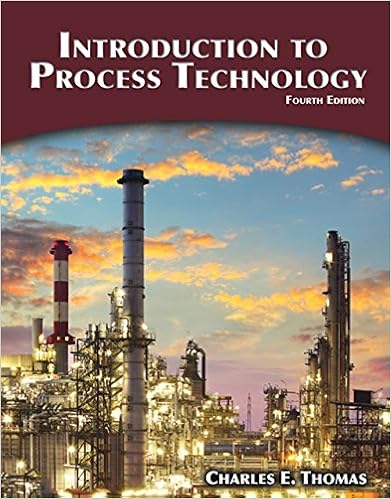# Increase which could be potentially fatal example a

• Notes
• 33
• 50% (2) 1 out of 2 people found this document helpful

This preview shows page 11 - 16 out of 33 pages.

##### We have textbook solutions for you!
The document you are viewing contains questions related to this textbook.The document you are viewing contains questions related to this textbook.
Chapter 4 / Exercise 6
Introduction to Process Technology
ThomasExpert Verified
increase which could be potentially fatal. Example: A sample of helium gas has a pressure of 2.50 atm and a volume of 125 mL. What will the volume be in mL if the pressure increases to 3.50 atm?Assume temperature and amount of gas remain constant. Summarize the problem:
##### We have textbook solutions for you!
The document you are viewing contains questions related to this textbook.The document you are viewing contains questions related to this textbook.
Chapter 4 / Exercise 6
Introduction to Process Technology
ThomasExpert Verified
Sarasua CHM 2045 Lecture Notes Tro Chapter 5 Sp2015 12 J.A.C. Charles 1787, a French mathematician and physicist observed the variation of volume and temperature when amount of gas was fixed and pressure was constant. Figure 5.10 p. 202 As T increases, gas expands (V increases). As T decreases, gas contracts (V decreases). You can see that all lines (data is linear) can be extrapolated to absolute zero of temperature 0.00K = -273.15ºC. There is a direct relationship between volume & temperature. V ∝ T or V=k T or 𝑉 𝑇 = k or 𝑉 𝑇 = constant For Charles’ Law, V and T vary while P,T and n are constant. Rearrange the Ideal gas Equation to get what changes (V and T) on left and what stays constant on right (P,n,R): PV=nRT ?𝑉 𝑇 =nR
Sarasua CHM 2045 Lecture Notes Tro Chapter 5 Sp2015 13 𝑉 𝑇 = ?𝑅 ? = constant A working relationship can be derived V 1 T 1 = V 2 T 2 T must be Kelvin; 1 and 2 correspond to initial and final conditions. (KMT) On a molecular level: heated gas molecules move faster, strike the container walls more frequently and with greater force so to keep the P constant, V must increase (density decreases) so that this motion is distributed over larger space. At lower T, molecules move more slowly so V decreases (density increases) to keep P constant. See Figure 5.11 p. 204. Example: A balloon has a volume of 5.0 L at room temperature (25.0 C). When placed in a freezer at -20.0 C, what will its new volume be in L? Assume pressure and amount of gas are constant. V 1 = 5.0L T 1 =25.0 C = 298.2K V 2 =? T 2 = -20.0 C = 253.2K V 1 T 1 = V 2 T 2
Sarasua CHM 2045 Lecture Notes Tro Chapter 5 Sp2015 So V 2 = V 1 T 1 x T 2 = V1 x T2 T 1 = 5.0? 𝑥 253.2? 298.2K = 4.2 L 3. Amonton’s Law or Gay- Lussac’s Law P,T vary at constant V, n The relationship between pressure and temperature is known as Amonton’s Law (end of 1600s) or Gay- Lussac’s Law (see p. 191). Pressure is directly related to kelvin T for a fixed amount of gas at constant V: P T or P=kT so ? 𝑇 = k or ? 𝑇 = constant PV = nRT so ? 𝑇 = ?𝑅 𝑉 = constant A working relationship can be derived P 1 T 1 = P 2 T 2 (KMT) From a molecular standpoint, at higher T, molecules strike the container walls more often and with greater force therefore pressure increases. Example: A sample of air in a closed 250.00 mL flask is heated from 20.0 C to 75.0 C. The pressure of the gas at 20.0 C = 0.98 atm. Calculate the pressure at 75.0 C.
14
Sarasua CHM 2045 Lecture Notes Tro Chapter 5 Sp2015 15 4. Avogadro’s Law : Volume and Amount (in Moles) Avogadro’s law assumes constant pressure and temperature. n,V vary at constant P,T It applies to all gases regardless of identity. See Figure 5.12 p. 189. It can be seen that 𝑉 ∝ n with a linear relationship. If moles
•••